# Community

Blog Events Webinars Tutorials Forum
×
Community Blog Exploring Deep Learning Models for Compression and Acceleration

# Exploring Deep Learning Models for Compression and Acceleration

Image compression and acceleration underpin most of the media applications in the consumer space. In this article, we will discuss how deep learning can improve these methods.

1. Compression and acceleration with deep learning models
1. Extremely Low Bit Neural Networks
2. Extremely Sparse Network
2. Training platforms
3. Highly efficient forward inference tools

## 1. Compression and Acceleration with Deep Learning Models

As the scale of deep learning networks increases, the computational complexity also increases accordingly, which severely limits its application to smart devices such as mobile phones. For example, the use of large-scale complex network models such as VGGNet and the residual network on an end device is not realistic.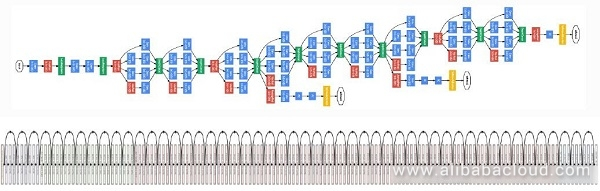Therefore, we need a deep learning model to perform compression and acceleration. We have described two major compression algorithms below.

### 1.1 Extremely Low Bit Neural Networks

The Low Bit model refers to compressing consecutive weights into discrete low-precision weights. As we have shown in the image below, the parameters of the original deep learning neural network are float variables and need 32 bit storage space. If we convert them into a state with only three values (0, +1, -1), then storage takes just 2 bits, significantly compressing the storage space and simultaneously avoiding multiplication operations. Only the symbol bit changes to addition and subtraction operations, thereby increasing computation speed.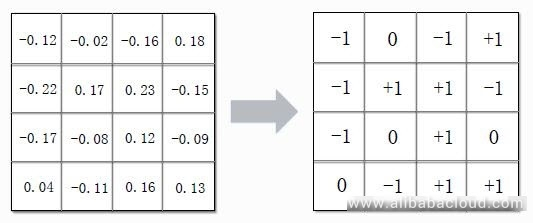Here is a reference article on Low Bit model - Extremely Low Bit Neural Networks: Squeeze the Last Bit Out with ADMM.

Next, we will use a binary network as an example to explain the above compression process. First, suppose that the optimized function of the original neural network is f(w), and the limiting condition is that the parameters of the deep learning network are constrained to within C. If C is (-1, 1), then the network is a binary network, as below: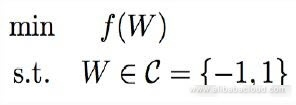Here, we will introduce an ADLM (Alternating Direction Method of Multipliers) that solves distributed optimization and constrained optimization to solve the above discrete non-convex constrained optimization problems. It takes the following form: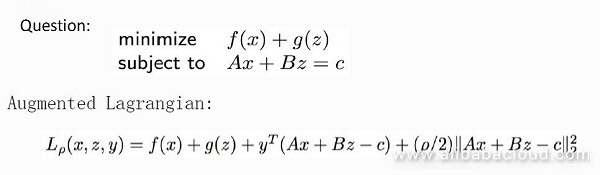We will use ADMM to solve when the objective function is f(x)+g(z), and where the constraint condition is the optimization of Ax+Bz=c. First, write the Augmented Lagrangian function, and then convert the above problem by solving xyz: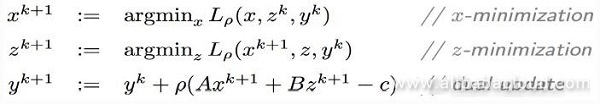That is, the minimum of xz is solved first, and then the updated value of y is obtained accordingly. The above is the ADMM standard solution. Next, let's see how we can convert the Low Bit Neural Network problem into an ADMM problem. First, we need to introduce the indicator function, the form of which is as follows: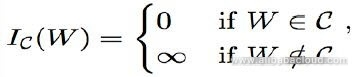Here, the objective function of the binary neural network is equivalent to the sum of the optimized objective function and the indicator function.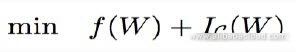This means that when the indicator function belongs to C, the optimization goal is equal to the initialization goal. When the current function does not belong to C, the indicator function is positive infinity, and the indicator function will be optimized first.

Then, we need to introduce a consistency restraint. Here we introduce the variable G and constrain W = G, so the objective function is equivalent to: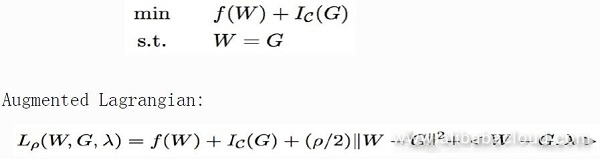After adding this helper variable, we can transform the optimization problem of the binary neural network into an issue of ADMM standard. Next, we write the formula for Augmented Langrangian and use the ADMM algorithm to reach the optimization goal, as we have described below: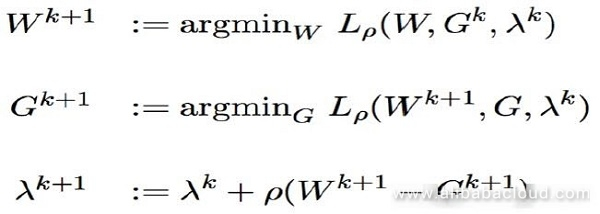Aside from the binary network described above, there are also the following common parameter spaces: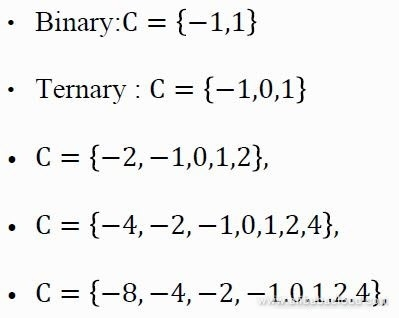After adding 2, 4, and 8 as values in the parameter space, there is still no need for multiplication. Instead, we need only the shift operations. Therefore, this replaces all multiplication operations in the neural network with shift and add operations.

We have shown the final optimization results after applying the above Low Bit model to ImageNet for classification in the following table: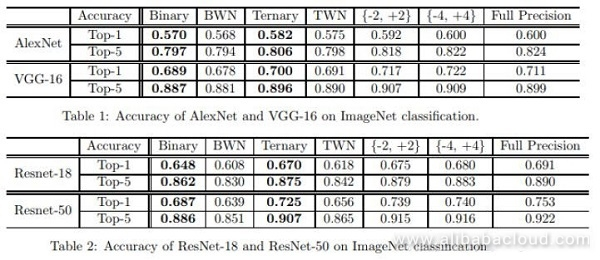Table 1 shows the application results of the algorithm in AlexNet and VGG-16. You will find that the algorithm is better in binary and trinary networks than in the original range. Furthermore, the classification results in the trinary network are nearly lossless when compared to results from the full precision classification. Table 2 shows the application of the algorithm in ResNet-18 and ResNet-50. The results are similar to those in Table 1.

In terms of detection, the algorithm still features high availability as you can see in the following table: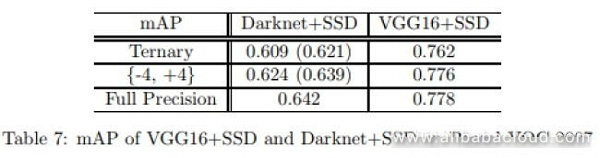We drew the data for this experiment from Pascal VOC 2007. According to the data in the above table, the accuracy of the detection results in the three-value space is almost negligible compared to the full-precision parameter space.

### 1.2 Extremely Sparse Networks

The sparse neural network is suitable for cases where most of the parameters in the network are zero. We can simplify the storage parameters using a simple compression algorithm, such as run-length coding, to reduce the parameter storage space significantly. Since we cannot involve 0 in the calculation, a large amount of computation space is saved, which greatly increases computation speed. In sparse networks, the optimization goal is still the same as above, but the restrictions get changed as follows: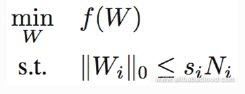We can obtain the falling gradient for f(w) and used it in successive iterations. In each iteration, we will perform connection pruning according to the standard that the smaller the W parameter, the less important it is. When we change small parameters to zero, we can maintain sparsity.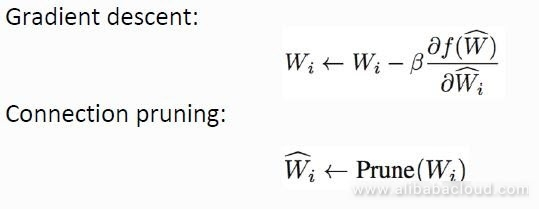However, there is an obvious problem with the above solution, as we have described in the following figure: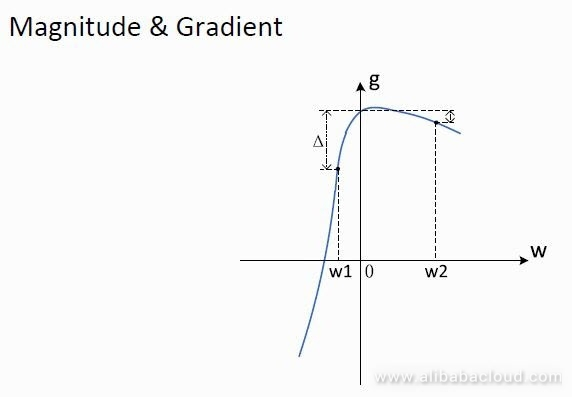W1 is closer to 0 than w2; however, if w1 is set to zero, the loss to the function is higher. Therefore, when determining the importance of w, consider the size and slope simultaneously. You can set it to zero only if the value of w and the slope are both low. Based on the above criteria, we conducted a rare experiment on Alexnet and GoogleNet, as we have shown below: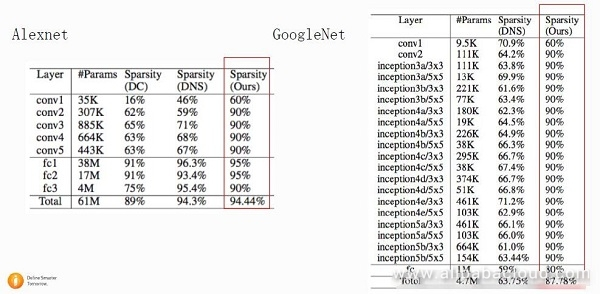We can see from the above image that whether it's a pure convolution network or it's inside a fully connected layer network, it can reach a sparsity of 90%.

### 1.3 Comparison of Experiment Results

We have described the sparse and quantized methods above. In Experiment 1, we have applied the two methods to Alexnet at the same time. The results are as follows: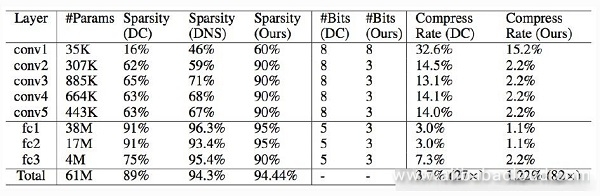We can see from the above image that at 3 Bits, when the sparsity is 90% or more, the loss of precision is almost negligible, with a compression rate of more than 82 times.

In Experiment 2, we applied both methods to ImageNet and Pascal VOC. Among them, P was sparse and Q was quantified. From the results in the figure, we can see that the accuracy loss of the experimental process was minimal, and the speed of inference in ImageNet was significantly improved. Pascal VOC can reach a sparsity of 88.7%, quantified in 3 bits with a compression ratio of 40 times. This is only a one-point drop from the full-precision network mAP.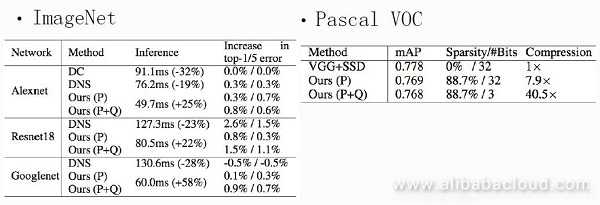## 2. Training Platforms

We established a Gauss training platform based on the above two methods. The Gauss training platform currently supports several common types of training tasks (like OCR, classification, and supervision) and models (like CNN and LSTM). It also supports the training of multiple machines and we can set it with the smallest number of parameters possible, reducing the amount of effort required from the user.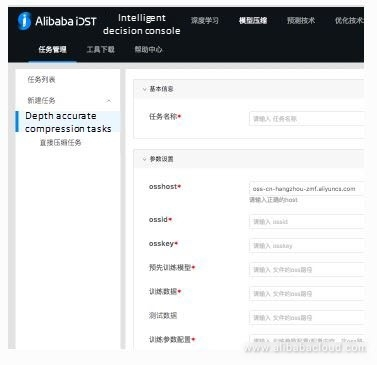At the same time, the Gauss training platform also supports two types of model training tools: Data-dependent and Data-independent. Data-dependent model training tools require users to provide training data. The training time is longer and it is suitable for scenarios that demand compression and acceleration. Data-independent training tools require no user-supplied training data and feature one-click processing and second-level processing.

## 3. Highly Efficient Forward Inference Tools

Even after the establishment of the training platform, the actual use of the model still requires efficient forward inference tools. We can implement Low-bit matrix calculation tools quickly using low-precision matrix calculation tools like AliNN and BNN. After implementation, the reasoning tool is 2-5 times faster than competing products on the ARM platform and three times faster on Intel platform.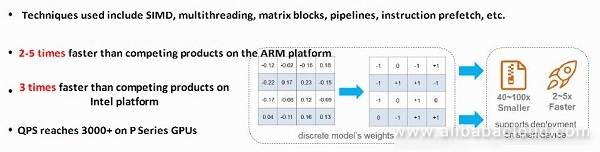## Author Bio

Li Xin is a senior algorithm expert in Alibaba's machine intelligence technology laboratory. He graduated from the Chinese Academy of Sciences with a Ph.D. in engineering and is committed to basic technology research in deep learning and its application in various industries.

0 0 0
Share on

# Related Products

• ##E-MapReduce Service

A Big Data service that uses Apache Hadoop and Spark to process and analyze data

• ##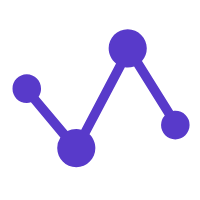MaxCompute

Conduct large-scale data warehousing with MaxCompute

• ##GPU(Elastic GPU Service)

Powerful parallel computing capabilities based on GPU technology.